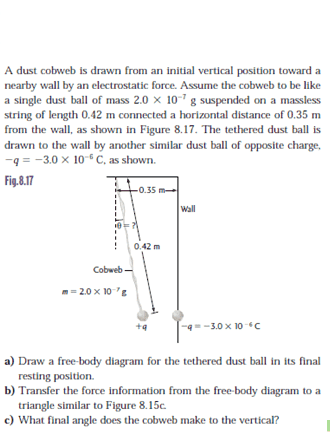# Electrostatic equilibrium, deflection of a cobweb

marcusesses

## Homework Statement## Homework Equations

After creating a FBD and analyzing the forces, I get the equation for the angle as

## tan \theta = \frac{k q^2}{m g r^2} ##

## The Attempt at a Solution

[/B]
I have tried to find a way to find the distance between the charges ## r ##, but the only way I can think of to find it required the angle. I do not know how the question can be solved without knowing at least one of the two variables, and my first inclination is just to assume there is not enough information.

Any help would be appreciated! Thanks!